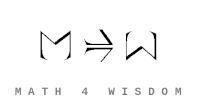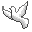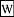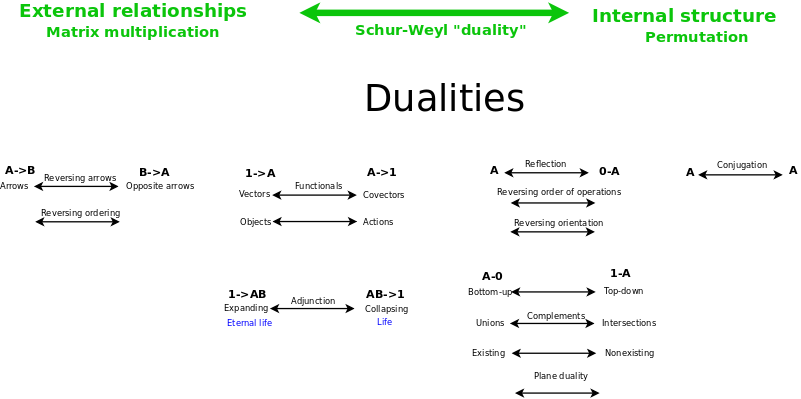Contact Andrius Kulikauskas ms@ms.lt +370 607 27 665 Eičiūnų km, Alytaus raj, LithuaniaMy original work is in the Public Domain for you to use in your best judgement. Support me! Patreon... in September? Paypal to ms@ms.ltBookshelfAs an Amazon Associate I earn from qualifying purchases. Thank you! Upload I am trying to understandSchur-Weyl duality. Why master this? Schur-Weyl duality is an overarching duality that unifies six other dualities.Schur Weyl duality relates the general linear group and the permutation group, thus relates: the duality of external relationships, where {$A\rightarrow B$} is dual to {$B\rightarrow A$}, as in matrix multiplication, where {$a_{ij}$} is dual to {$a_{ji}$} the duality of internal structure, where conjugates {$i$} and {$j$} are indistinguishable and of equal significance, as with permutations. How to learn this? Some related concepts to learn are:Category of representations. In this category, the morphisms are theequivariant maps.Frobenius reciprocity This is an adjunction between the functor {$\textrm{Res}^G_H$} restricting a linear representation of a group {$G$} to a linear representation of its subgroup {$H$} and the functor {$\textrm{Ind}^G_H$} inducing a linear representation of {$G$} from a linear representation of {$H$}. This adjunction {$\textrm{Ind}^G_H \dashv\textrm{Res}^G_H$} is of additional interest because if {$G$} and {$H$} are finite groups, then also {$\textrm{Res}^G_H \dashv\textrm{Ind}^G_H$}. I want to understand this adjunction from both sides.Maschke's theorem states that every representation of a finite group G over a field F with characteristic not dividing the order of G is a direct sum of irreducible representations. It seems this theorem makes use of the concepts of restriction and induction. I am also interested in the module theoretic version of this theorem because it is related toWedderburn-Artin theorem which describes how semisimple rings are products of finite-dimensional matrix rings over division rings. The latter theorem is used in theclassification of Clifford algebras and thus is relevant for Bott periodicity. I am watching a helpful series of videos by Monica Vazirani: Representation Theory and Combinatorics of the Symmetry Group and Related Structures, Part II, Part III.
Šis puslapis paskutinį kartą keistas August 05, 2021, at 07:42 PM